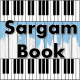# Beer Never Broke My Heart Sargam Notes Luke Combs

Beer Never Broke My Heart Sargam Notes Luke Combs Available On Sargam Book. Beer Never Broke My Heart Easy Harmonium Notes For Beginners. Simple Ways To Learn.

Song : Beer Never Broke My Heart
Singer : Luke Combs
Western Notes : Website 1, Website 2, Website 3
Classical Sargam Notes : www.sargambook.com
Guitar / Piano Chords : https://guitar.pianodaddy.com

I’ve had a largemouth bass bust my line
Ma Dha(k) Ma, Dha(k) Ma Dha(k)
Dha(k) Ni(k) Dha(k), Dha(k) Dha(k)
A couple beautiful girls tell me goodbye
Dha(k) Dha(k), Ni(k) Dha(k) Ma Dha(k)
Ma Ga(k), Ma Ga(k) Re(k) Re(k)
Trucks break down, dogs run off
Dha(k) Ni(k) Dha(k) Dha(k)
Dha(k) Ni(k) Dha(k) Dha(k) Ma
Politicians lie, been fired by the boss
Dha(k) Ni(k) Dha(k), Dha(k) Ma Dha(k) Ma
Ga(k) Ma Ga(k) Re(k), Re(k) Re(k) Re(k)

It takes one hand
Re(k) Re(k), Ga(k) Ma Dha(k)
Ga(k) Ma
To count the things I can count on
Re(k) Re(k) Re(k) Ga(k)
Ga(k) Ma Dha(k), Ga(k) Ma
No, there ain’t much, man
Re(k) Re(k) Re(k), Re(k) Ga(k)
Ma Dha(k) Ga(k) Ma
That ain’t never let me down
dha(k) Ma Dha(k) Ma, Ma Ga(k)
Ga(k) Re(k) Re(k)

Longneck ice-cold beer never broke my heart
GA(k) MA, GA(k) MA, GA(k) MA, GA(k) MA
RE(k) Ni(k) RE(k), GA(k) RE(k) Ni(k) Dha(k)
Like diamond rings and football teams have torn this boy apart
RE(k) RE(k), GA(k) MA MA, GA(k) MA, GA(k) MA GA(k) RE(k) RE(k)
RE(k) Ni(k) GA(k) GA(k), GA(k) RE(k) GA(k) MA
Like a neon dream, it just dawned on me that bars and this guitar
GA(k) RE(k), GA(k) MA MA GA(k) MA, MA MA GA(k) RE(k) RE(k)
RE(k) MA(t), MA(t) MA GA(k) RE(k) RE(k)
And longneck ice-cold beer never broke my heart
GA(k) MA, GA(k) MA, GA(k) MA, GA(k) MA
RE(k) GA(k) GA(k) GA(k), MA GA(k) RE(k) RE(k)

These are demo notes for respective song. You can try it on your instrument. If it works for you and you are comfortable to play along with our notes, you can simply get full notes by paying us. Just click the Buy Now button below and see our packages.#### Titiksha

Titiksha Telang is an Indian Musician & Singer. She is MA in Indian Classical Music & Owner of Sargam Book Website.